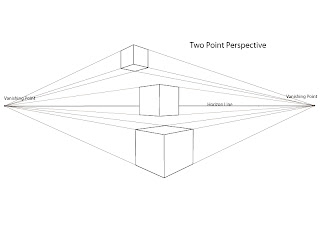## Pages

### Drawing Basics: Two Point Perspective

Today I thought I would discuss the basics of two point perspective and how to draw a cube using this. Two point perspective is a useful guide to help create three-dimensional scenes on a two-dimensional surface.

Lets figure out how to draw in two point perspective, but before we do, lets review some key terms discussed in the post about one point perspective.

The horizon line is the eye level of the viewer and runs on a horizontally across the scene. This line sets up the orientation of the scene and gives the viewer some bearing as to how the visually relate to the image.

Vanishing points are the points on the horizon line where perceptually all things converge. In two point perspective, the objects appear to diminish they follow path back to points on the far left and right of the horizon line.

Orthogonal lines are the lines that move from the object to the vanishing points. The purpose of these lines is to set up the visual path to a vanishing point, directing the shape of the object in perspective. Using these lines, everything in the scene will converge at the vanishing points.

Two Point Perspective

As the name suggests two point perspective takes advantage of two vanishing points rather than just one. This allows for more flexibility with a scene as the front plane of the object does not have to face the viewer directly.

To explain two point perspective, lets start by drawing a cube using two point perspective as a guide.

First you need to draw a horizon line.

Second determine where the vanishing points will be placed on the horizon line. Be careful to keep the points  far enough apart to avoid distortion as placing them to close together will create skewed edges of an object.
Next draw the leading edge of the cube. This would be the closest corner edge of the two side planes on the cube facing you.

Then draw the orthogonal lines from the top and bottom of the leading edge back to both the vanishing points.

Draw the trailing edges of the cube (the back edges of the two side planes) between the orthogonal lines. Don't worry about how to get them in the exact place right now, I will demonstrate that later. For now, a good estimate will do.

Next draw the orthogonal lines from the top of the trailing edges. Start by drawing a line from top left edge to the right vanishing point. Then draw from the right edge to the left vanishing point. The two lines should intersect and form the back corner of the top plane of the cube.

Now the cube is formed, to the left you can see that top and bottom edges of the cube are created by the orthogonal lines and the side edges are perpendicular to the horizon line. Again, remember that the orthogonal lines are just guides, the edges of more organic shapes will not follow the orthogonal line exactly.

There you have it.  A cube drawn in perspective. The object appears to be three dimensional and sits in a space.Cubes in two point perspective.
In two point perspective, vertical edges are always perpendicular to the horizon line. Also, the relative size of an object will diminish the further back in the scene it is placed. This means that taking two objects the same size and placing them at different distances within the scene, they will appear different in relative size.

Notice how the orthogonal lines coming from objects above the horizon line drop down to the vanishing points. This allows us to see the bottom of the cube floating above the horizon line. The opposite is true for the orthogonal lines for objects below the horizon line, they rise up to the vanishing points. This allows for us to see the top plane of the cube that is below the horizon line.

Notice how you can not see either the top or the bottom plane of the cube intersecting the horizon line. This is the leveling effect of the horizon line, the closer an object moves towards the horizon line the top and bottom planes will begin to flatten. If the edge is directly on the horizon line it will appear horizontal and match the horizon line.

You can use two point perspective a a guide to set up scenes that give the illusion of three dimensional space. There is more to two point perspective, but this will give you a good starting point.

I recommend after doing this exercise find a object the shape of a cube or roughly the shape of a cube and practice drawing it in perspective, by applying the principles you have learned to the cube you see in front of you. This will help you understand how to determine where the horizon line is in relationship to your cube, figure out where everything converges on that horizon line to place the vanishing points, and practice drawing shape in perspective.

1.2.3.